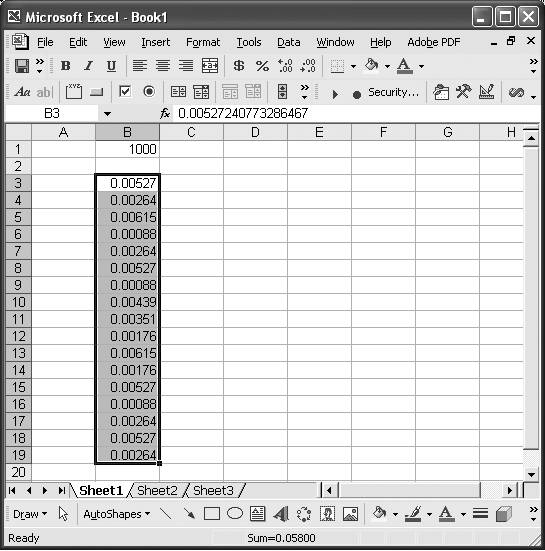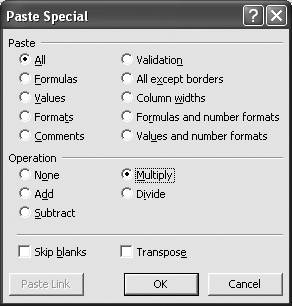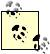# Mastering Multiplication

Problem

You already know how to perform normal multiplication using the * operator, but need to perform other multiplication operations.

Solution

Use Excel's various multiplication functions , which are summarized in Table 7-3.

Table 7-3. Common multiplication functions

Function

Syntax

Description

PRODUCT

=PRODUCT(n1, n2, n3, ...)

Returns the product of all given numbers, which may include cell ranges and explicit numbers.

LCM

=LCM(n1, n2, n3, ...)

Returns the least common multiple of the given integer numbers.

SUMPRODUCT

=SUMPRODUCT(array1, array2,...)

Adds the products of corresponding values in given cell ranges (called arrays). The ranges must have the same number of elements.

Discussion

Excel provides a handy utility to multiply a range of numbers by some value in one fell swoop. For example, say you have a range of data like that shown in Figure 7-1, whch you'd like to scale by a factor of 1,000.

Figure 7-1. Data to be scaledFollow these steps to quickly scale the data in the range B3:B19 by 1,000. Type the scale factor, 1000 in this case, in a cell (in Figure 7-1, I put it in cell B1). Select the cell containing the scale factor. Select Edit [Image_Link]/images/U2192.jpg border=0> Copy from the main menu bar. Now, with the mouse, select the range containing the data to be scaled (range B3:B19 in this example). From the main menu bar, select Edit [Image_Link]/images/U2192.jpg border=0> Paste Special... to open the Paste Special dialog box shown in Figure 7-2.

Figure 7-2. Paste Special dialog boxNow select the Multiply option and press the OK button. The selected data will be multiplied by the scale factor in place; i.e., the scaled data will replace the original data.Excel will revise the formulas to include the scale factor if the cells to be scaled contain formulas instead of values. For example, if cell B3 in the example discussed here contained the formula =A3+A4, then after scaling it would contain the formula =(A3+A4)*1000.

You'll notice from Figure 7-2 that Paste Special also provides other operations including adding, subtracting, and dividing. You can use the same technique with these other operations to conveniently shift or scale data.

Excel provides support for matrix multiplication and complex number multiplication. See Recipes 7.10 and 7.13 for more information.Excel Scientific and Engineering Cookbook (Cookbooks (OReilly))
ISBN: 0596008791
EAN: 2147483647
Year: N/A
Pages: 206
Authors: David M Bourg